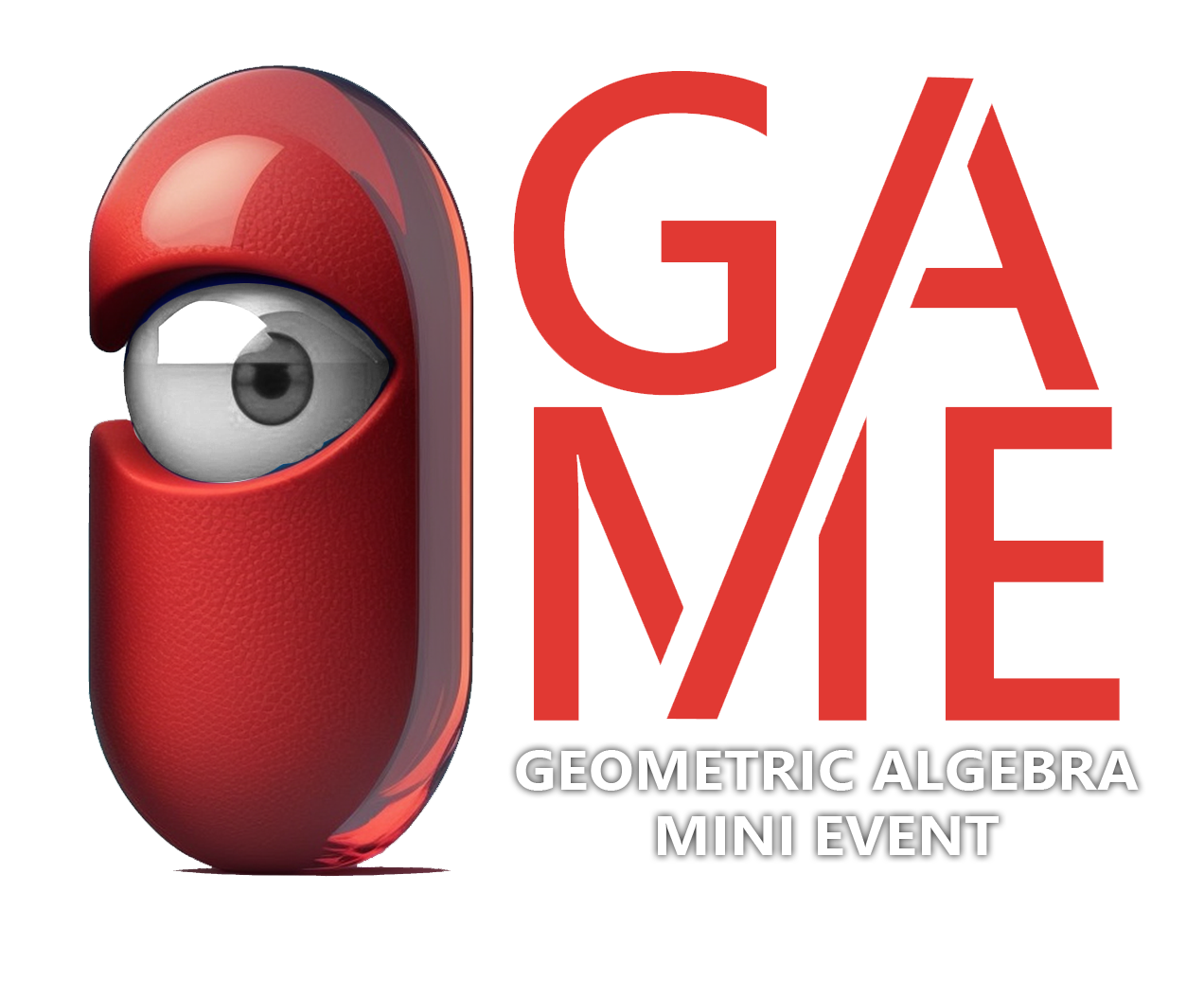GAME2023 - Geometric Algebra Mini Event.
From the 19th to the 21st of April, Kortrijk (Belgium) is transformed into a true temple of geometry !

### Featured Introductions

Geometric Numbers
SIGGRAPH 2019 Geometric Algebra
Geometric Algebra

Clifford's Geometric Algebra enables a unified, intuitive and fresh perspective on vector spaces, giving elements of arbitrary dimensionality a natural home.

### The Vector

The Vector is an oriented, one dimensional
quantity. Two $\parallel$ Vectors multiply to a Scalar ($\mathbb R$).
Two $\perp$ vectors anti-commute ($e_1e_2=-e_2e_1$)

### The Bivector

The Bivector is an oriented, two dimensional
quantity. Bivectors naturally represent
transformations. Similarly, $n$ vectors combine into an $n$-vector.

The $n$-dimensional geometric algebra $\mathbb R_{p,q,r}$ is constructed from $p$ positive, $q$ negative and $r$ null vectors called generators, written $\bf e_i$

$\mathbb R$

### The Scalar

The Scalars $\mathbb R$ are included in
the algebras. every basis $n$-vector
squares to a Real Number.

$e^{\mathbf e_{ij}}$

### The Rotor

The product of two vectors, or the
exponentiation of a bivector creates
a rotor. (rotation, translation, ..)

A generic element of the algebra is called a multivector and is a linear combination of scalar, vector and $n$-vector parts. $$\mathbf X = \alpha_0 + \alpha_1 \mathbf e_1 + .. + \alpha_i \mathbf e_{12} + .. + \alpha_n \mathbf e_{12..n}$$

### Vectorspace Geometric Algebra

The Geometric Algebras for the 2D and 3D vectors naturally include 2D and 3D rotations.

$\mathbb R_2^+ \cong \mathbb C$

### 2D Vectors

The geometric algebra $\mathbb R_2$ of the
2D Vectors has the complex numbers
as its even subalgebra.

$\mathbb R_3^+ \cong \mathbb H$

### 3D Vectors

The geometric algebra $\mathbb R_3$ of the
3D Vectors has the quaternions
as its even subalgebra.

### Projective Geometric Algebra

Using one extra dimension, we obtain the plane-based Projective Geometric Algebra for 2 and 3 dimensions. Its elements are points, lines and planes. It includes elements at infinity and has exception free join and meet operations. Its even subalgebra provides in rotations & translations and is isomorphic to the dual quaternions

### $\mathbb R^*_{2,0,1}$ : 2D PGA

In 2D PGA points join $\vee$ into
lines and lines meet $\wedge$ into points.
Rotations and translations are unified,
with bivectors isomorphic to $\mathfrak{SE}(2)$

### $\mathbb R^*_{3,0,1}$ : 3D PGA

In 3D PGA points and lines join $\vee$ into
lines and planes, while lines and planes meet $\wedge$
into points and lines. The bivectors are isomorphic
to $\mathfrak{SE}(3)$, the dual quaternions

### Conformal Geometric Algebra

Using two extra dimensions, we obtain the point-based Conformal Geometric Algebra for 2 and 3 dimensions. Its elements are points, point pairs, lines, circles, spheres and planes. It includes an infinite point and has exception free join and meet operations. Its even subalgebra provides in conformal transformations.

### $\mathbb R_{3,1}$ : 2D CGA

In 2D CGA, points join ($\wedge$) into lines
and circles, and lines and circles meet ($\vee$) in points.
Rotations, Translations and Dilations all
come in versor form.

### $\mathbb R_{4,1}$ : 3D CGA

In 3D CGA, points join ($\wedge$) into pairs, lines,
circles, planes and spheres, which meet ($\vee$) in points,
pairs, circles and lines. Rotations, Translations
and Dilations all come in versor form.